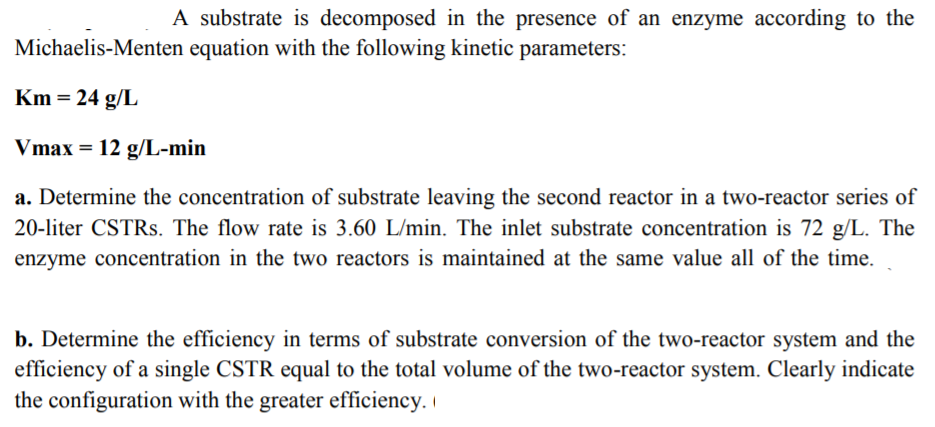A substrate is decomposed in the presence of an enzyme according to theMichaelis-Menten equation with the following kinetic parametersKm-24 g/LVmax 12 g/L-mina. Determine the concentration of substrate leaving the second reactor in a two-reactor series of20-liter CSTRs. The flow rate is 3.60 L/min. The inlet substrate concentration is 72 g Theenzyme concentration in the two reactors is maintained at the same value all of the time.b. Determine the efficiency in terms of substrate conversion of the two-reactor system and theefficiency of a single CSTR equal to the total volume of the two-reactor system. Clearly indicatethe configuration with the greater efficiency.i

Question

PLease show all stepshelp_outlineImage TranscriptioncloseA substrate is decomposed in the presence of an enzyme according to the Michaelis-Menten equation with the following kinetic parameters Km-24 g/L Vmax 12 g/L-min a. Determine the concentration of substrate leaving the second reactor in a two-reactor series of 20-liter CSTRs. The flow rate is 3.60 L/min. The inlet substrate concentration is 72 g The enzyme concentration in the two reactors is maintained at the same value all of the time. b. Determine the efficiency in terms of substrate conversion of the two-reactor system and the efficiency of a single CSTR equal to the total volume of the two-reactor system. Clearly indicate the configuration with the greater efficiency.i fullscreen
Step 1

Part (a)

Let the reaction taking place in the CSTR be,

S→P

According to the Michaelis-Menten equation, the reaction rate for this reaction is defined as written below.

Step 2

For two CSTR’s in series;

Volume of each CSTR is, V = 20 L

Volumetric flowrate is, V̇ = 3.60 L/min

Initial concentration of the substrate is, [S0] = 72 g/L

Step 3

Now, calculate the convers...

Want to see the full answer?

See Solution

Want to see this answer and more?

Our solutions are written by experts, many with advanced degrees, and available 24/7

See Solution
Tagged in

Chemical Technology